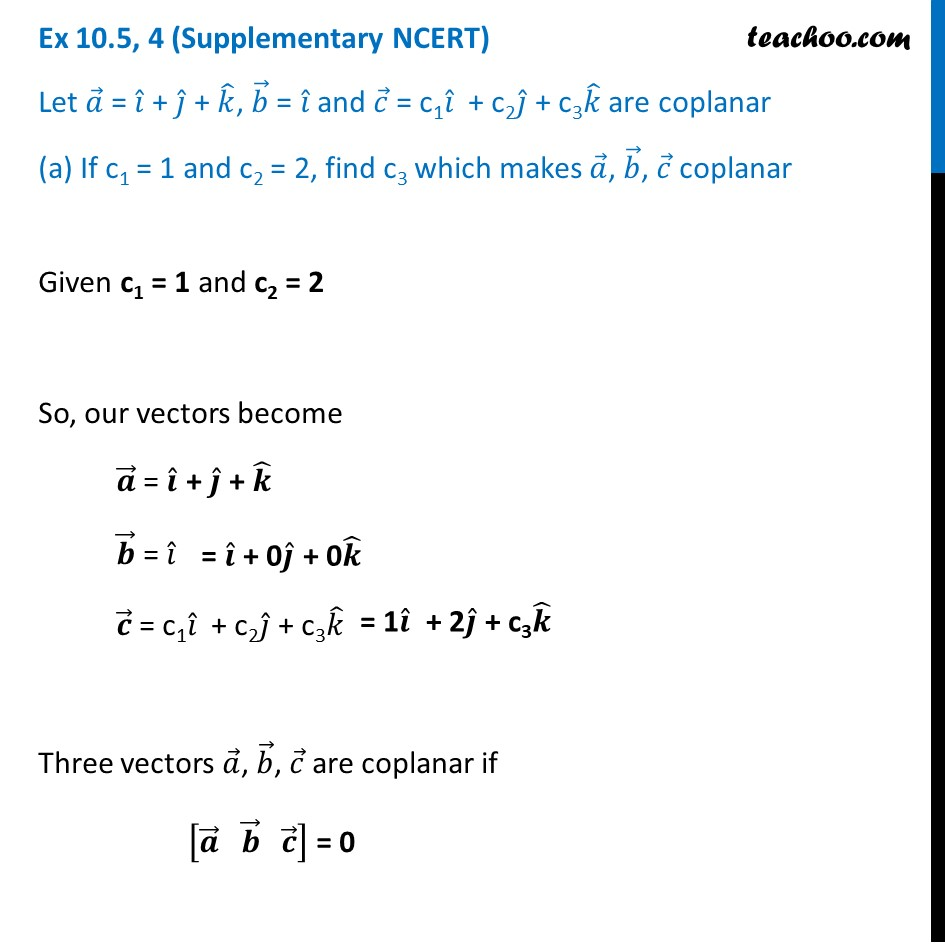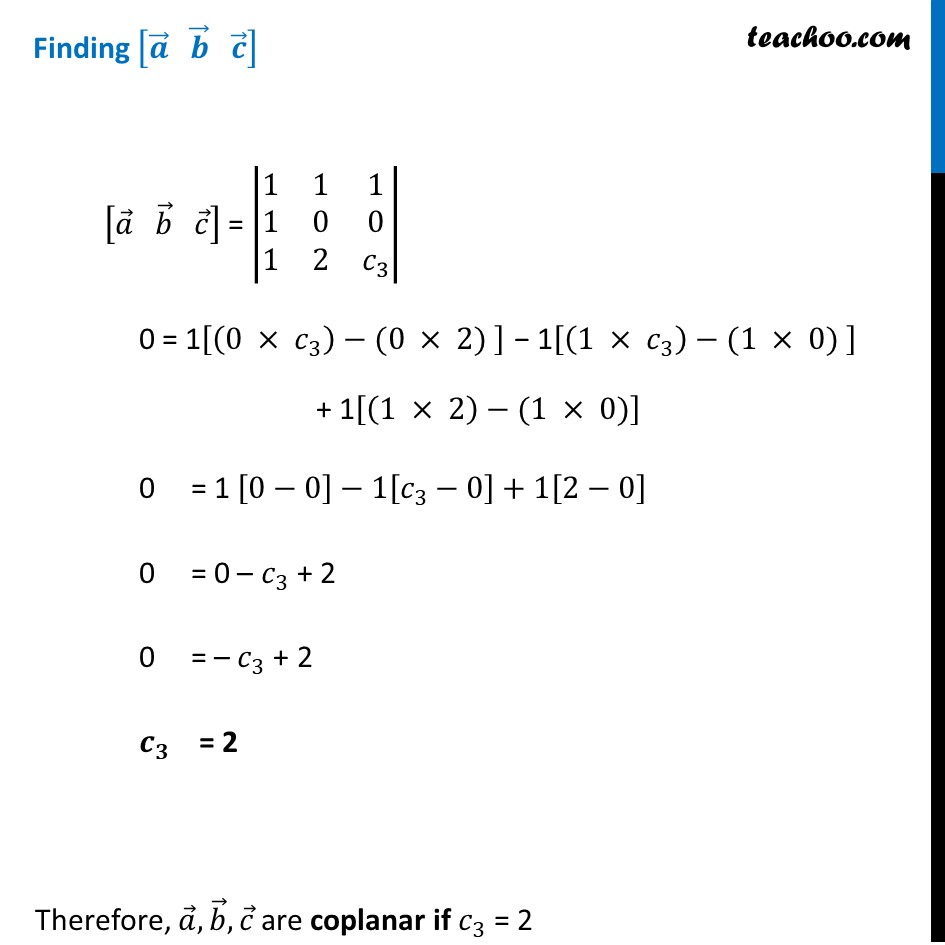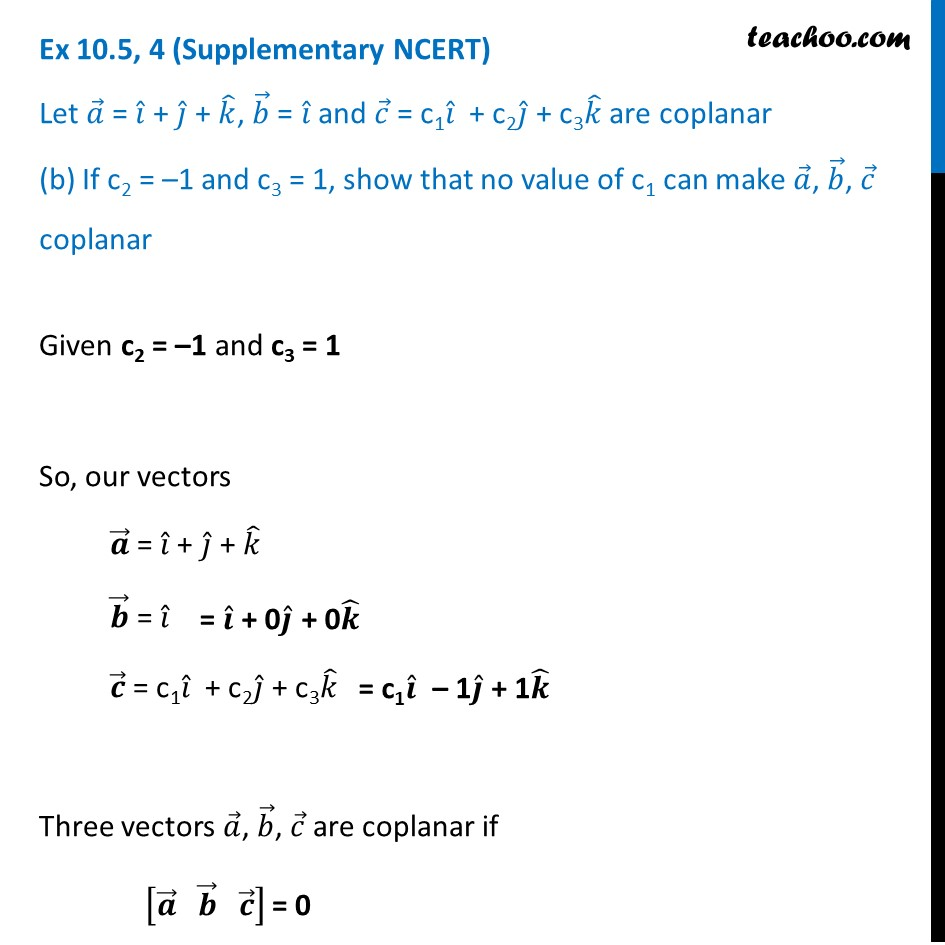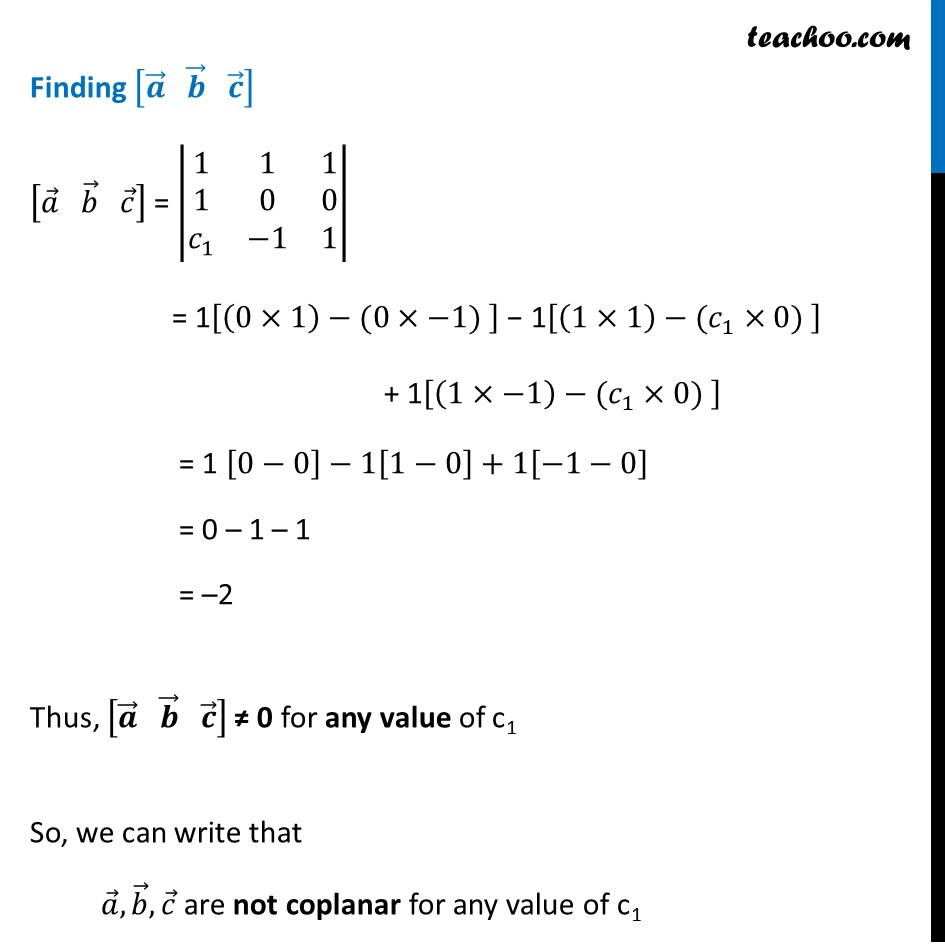Ex 10.5 (Supplementary NCERT)

Chapter 10 Class 12 Vector Algebra
Serial order wiseLearn in your speed, with individual attention - Teachoo Maths 1-on-1 Class

### Transcript

Ex 10.5, 4 (Supplementary NCERT) Let 𝑎 ⃗ = 𝑖 ̂ + 𝑗 ̂ + 𝑘 ̂, 𝑏 ⃗ = 𝑖 ̂ and 𝑐 ⃗ = c1𝑖 ̂ + c2𝑗 ̂ + c3𝑘 ̂ are coplanar (a) If c1 = 1 and c2 = 2, find c3 which makes 𝑎 ⃗, 𝑏 ⃗, 𝑐 ⃗ coplanar Given c1 = 1 and c2 = 2 So, our vectors become 𝒂 ⃗ = 𝒊 ̂ + 𝒋 ̂ + 𝒌 ̂ 𝒃 ⃗ = 𝑖 ̂ 𝒄 ⃗ = c1𝑖 ̂ + c2𝑗 ̂ + c3𝑘 ̂ Three vectors 𝑎 ⃗, 𝑏 ⃗, 𝑐 ⃗ are coplanar if [𝒂 ⃗" " 𝒃 ⃗" " 𝒄 ⃗ ] = 0 Ex 10.5, 4 (Supplementary NCERT) Let 𝑎 ⃗ = 𝑖 ̂ + 𝑗 ̂ + 𝑘 ̂, 𝑏 ⃗ = 𝑖 ̂ and 𝑐 ⃗ = c1𝑖 ̂ + c2𝑗 ̂ + c3𝑘 ̂ are coplanar (b) If c2 = –1 and c3 = 1, show that no value of c1 can make 𝑎 ⃗, 𝑏 ⃗, 𝑐 ⃗ coplanar Given c2 = –1 and c3 = 1 So, our vectors 𝒂 ⃗ = 𝑖 ̂ + 𝑗 ̂ + 𝑘 ̂ 𝒃 ⃗ = 𝑖 ̂ 𝒄 ⃗ = c1𝑖 ̂ + c2𝑗 ̂ + c3𝑘 ̂ Three vectors 𝑎 ⃗, 𝑏 ⃗, 𝑐 ⃗ are coplanar if [𝒂 ⃗" " 𝒃 ⃗" " 𝒄 ⃗ ] = 0 Finding [𝒂 ⃗" " 𝒃 ⃗" " 𝒄 ⃗ ] [𝑎 ⃗" " 𝑏 ⃗" " 𝑐 ⃗ ] = |■8(1&1&1@1&0&0@𝑐_1&−1&1)| = 1[(0×1)−(0×−1) ] − 1[(1×1)−(𝑐_1×0) ] + 1[(1×−1)−(𝑐_1×0) ] = 1 [0−0]−1[1−0]+1[−1−0] = 0 – 1 – 1 = –2 Thus, [𝒂 ⃗" " 𝒃 ⃗" " 𝒄 ⃗ ] ≠ 0 for any value of c1 So, we can write that 𝑎 ⃗,𝑏 ⃗,𝑐 ⃗ are not coplanar for any value of c1